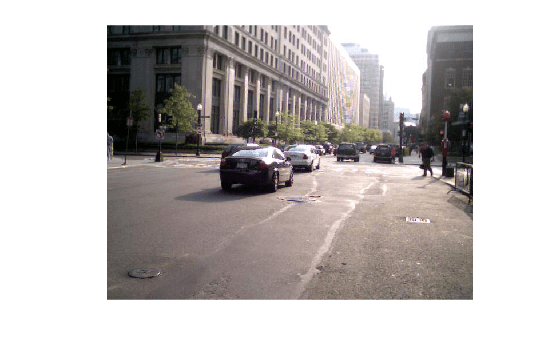Documentation

Integer Arithmetic

This example shows how to perform arithmetic on integer data representing signals and images.

Load Integer Signal Data

Load measurement datasets comprising signals from four instruments using 8 and 16-bit A-to-D's resulting in data saved as int8, int16 and uint16. Time is stored as uint16.

% Look at variables
whos Signal1 Signal2 Signal3 Signal4 Time1
Name            Size            Bytes  Class     Attributes

Signal1      7550x1              7550  int8
Signal2      7550x1              7550  int8
Signal3      7550x1             15100  int16
Signal4      7550x1             15100  uint16
Time1        7550x1             15100  uint16

Plot Data

First we will plot two of the signals to see the signal ranges.

plot(Time1, Signal1, Time1, Signal2);
grid;
legend('Signal1','Signal2');Here we see the values for int8. It is likely that these values would need to be scaled to calculate the actual physical value that the signal represents e.g. Volts.

Process Data

We can perform standard arithmetic on integers such as +, -, *, and /. Let's say we wished to find the sum of Signal1 and Signal2.

SumSig = Signal1 + Signal2; % Here we sum the integer signals.

Now let's plot the sum signal and see where it saturates.

cla;
plot(Time1, SumSig);
hold on
Saturated = (SumSig == intmin('int8')) | (SumSig == intmax('int8')); % Find where it has saturated
plot(Time1(Saturated),SumSig(Saturated),'rd')
grid
hold offThe markers show where the signal has saturated.

Load Integer Image Data

Next we will look at arithmetic on some image data.

street1 = imread('street1.jpg'); % Load image data
whos street1 street2
Name           Size                Bytes  Class    Attributes

street1      480x640x3            921600  uint8
street2      480x640x3            921600  uint8

Here we see the images are 24-bit color, stored as three planes of uint8 data.

Display Images

Display first image.

cla;
image(street1); % Display image
axis equal
axis offDisplay second image

image(street2); % Display image
axis equal
axis offScale an Image

We can scale the image by a double precision constant but keep the image stored as integers. For example,

duller = 0.5 * street2; % Scale image with a double constant but create an integer
whos duller
Name          Size                Bytes  Class    Attributes

duller      480x640x3            921600  uint8
subplot(1,2,1);
image(street2);
axis off equal tight
title('Original');  % Display image

subplot(1,2,2);
image(duller);
axis off equal tight
title('Duller');    % Display imageWe can add the two street images together and plot the ghostly result.

combined = street1 + duller; % Add |uint8| images
subplot(1,1,1)
cla;
image(combined); % Display image
title('Combined');
axis equal
axis off